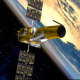# Gmapping and CPU load [closed]Hi all,

I'm using Gmapping to create a map with an hokuyo lx30 laser. I have created a bag file of around 20 minutes of laser scans in an indoor environment, to run Gmapping offline.

When running in the robot's Intel Atom Dual Core, it produces almost 400 scan failures, and the resulting map is really terrible.

When running in my Dual Core desktop, it produces 50 scan failures, and the resulting map is quite acceptable.

What is really surprising is that the CPU load of the Intel Atom is much lower (5% in only one of the 4 CPUs) that that of the desktop (14% in 2 of the 4 CPUs). I have tried to run rosbag play 10 times slower, and so doing both machines generate acceptable maps, dropping the failed scans to 10 and 7 in each case. Interestingly, the CPU loads remained constant in both experiments.

So it looks like Gmapping gently rejects to use the whole CPU but generates a terrible map?

Maps comparison: C:\fakepath\compare_maps.jpg

The parameters used (much more "generous" than the default ones) are:

<param name="throttle_scans" value="1" />
<param name="base_frame" value="/base_footprint" />
<param name="odom_frame" value="/odom" />
<param name="map_frame" value="/map" />
<param name="map_udpate_interval" value="2.5"/>
<param name="maxUrange" value="5.5"/>
<param name="maxRange" value="5.8"/>
<param name="sigma" value="0.05"/>
<param name="kernelSize" value="1"/>
<param name="lstep" value="0.05"/>
<param name="astep" value="0.05"/>
<param name="iterations" value="5"/>
<param name="lsigma" value="0.125"/>
<param name="ogain" value="3.0"/>
<param name="lskip" value="0"/>
<param name="srr" value="0.06"/>
<param name="srt" value="0.12"/>
<param name="str" value="0.08"/>
<param name="stt" value="0.12"/>
<param name="linearUpdate" value="0.3"/>
<param name="angularUpdate" value="0.15"/>
<param name="temporalUpdate" value="-1.0"/>
<param name="resampleThreshold" value="0.5"/>
<param name="particles" value="100"/>
<param name="xmin" value="-50.0"/>
<param name="ymin" value="-50.0"/>
<param name="xmax" value="50.0"/>
<param name="ymax" value="50.0"/>
<param name="delta" value="0.08"/>
<param name="llsamplerange" value="0.01"/>
<param name="llsamplestep" value="0.01"/>
<param name="lasamplerange" value="0.005"/>
<param name="lasamplestep" value="0.005"/>


But I have tried also with Gmapping default parameters and the results are comparable.

Any clues? I'm really puzzled. Btw, I use ROS electric on Ubuntu Lucid. Thanks

edit retag reopen merge delete